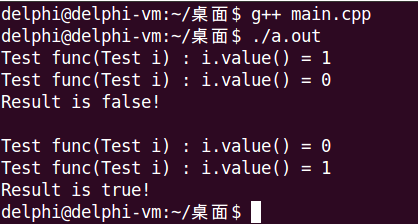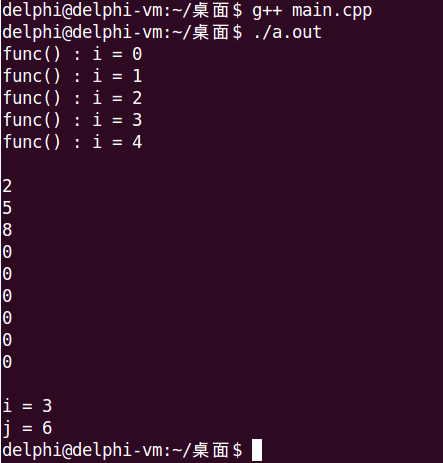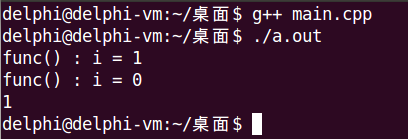Categories

1. Conclusion

1. Conclusion

The two previous notes are in C ++ can overload operators and without side effects, this notebook is rather special, mainly lists two C ++ syntax allows overloading, but should not be (allowed) in the project overloaded operators :

Logical operators && and ||

Comma operator, comma expression composed of

The reason these two operators are not allowed in the project is overloaded: native semantics not be fully realized after the operator overloading.

First recall logical operators native semantics

Operands only two kinds of true and false values

Does not require complete logical expression can be calculated to determine the final result (shorted law)

The end result can only be true or false

C ++ syntax is to allow overloading logical operators, see the following sample code

``````#include
#include

using namespace std;

class Test
{
int mValue;
public:
Test(int v)
{
mValue = v;
}
int value() const
{
return mValue;
}
};

bool operator && (const Test &l, const Test &r)
{
return l.value() && r.value();
}

bool operator || (const Test &l, const Test &r)
{
return l.value() || r.value();
}

Test func(Test i)
{
cout << "Test func(Test i) : i.value() = " << i.value() << endl;

return i;
}

int main()
{
Test t0(0);
Test t1(1);

/*
* 根据短路法则，期望的正确输出为:
* Test func(Test i) : i.value() = 0
* Result is false!
*/
if( func(t0) && func(t1) )
{
cout << "Result is true!" << endl;
}
else
{
cout << "Result is false!" << endl;
}

cout << endl;

/*
* 根据短路法则，期望的正确输出为:
* Test func(Test i) : i.value() = 1
* Result is true!
*/
if( func(1) || func(0) )
{
cout << "Result is true!" << endl;
}
else
{
cout << "Result is false!" << endl;
}

return 0;
}``````We look forward to the comments in the code of the correct output, but the actual running of the results of a failed, why?

Essence operator overloading is extended by the function call operator functions, if the condition lines 47 and 63 are respectively equivalent to the line operator && (func (t0), func (t1)) and the operator || (func (t1), func (t0))

Overloaded functions calculated for all parameters must be completed before entering the body of the function, the function calculation order parameter is uncertain

Shorting complete failure rule, not be fully realized after the logical semantics native operator overloading

Comma operator, may constitute comma expression, the following expression is native semantic comma

Comma expression for connecting a plurality of sub-expressions of an expression, such as exp1, exp2, exp3, ..., expN

Commas before N-1 expression subexpression can not return a value, and finally a sub-expression must have a return value

The final result of the comma expression is the last expression of the value of a child

Comma expression strictly calculated for each sub-expression in left to right order

``````#include
#include

using namespace std;

void func(int i)
{
cout << "func() : i = " << i << endl;
}

int main()
{
int a =
{
(0, 1, 2),   //逗号表达式，等价于a = {2, 5, 8,};
(3, 4, 5),
(6, 7, 8)
};

int i = 0;
int j = 0;

while (i < 5)
func(i),    //逗号表达式，等价于func(i), i++;

i++;

cout << endl;

for (i = 0; i < 3; i++)
{
for (j = 0; j < 3; j++)
{
cout << a[i][j] << endl;
}
}

cout << endl;

(i, j) = 6;  //逗号表达式，等价于 j = 6;

cout << "i = " << i << endl;
cout << "j = " << j << endl;

return 0;
}``````Overload comma operator in C ++ is legal, the parameters of overloaded functions must be a class type, and the type of the return value must be a reference overloaded functions

``````Class &operator , (const Class &a, const Class &b)
{
return const_cast(b);
}``````

See the following sample code

``````#include
#include

using namespace std;

class Test
{
int mValue;
public:
Test(int i)
{
mValue = i;
}
int value()
{
return mValue;
}
};

Test &operator , (const Test &a, const Test &b)
{
return const_cast(b);
}

Test func(Test &i)
{
cout << "func() : i = " << i.value() << endl;

return i;
}

int main()
{
Test t0(0);
Test t1(1);

/*
* 期望的正确输出为:
* func() : i = 0
* func() : i = 1
* 1
*/
Test tt = (func(t0), func(t1));
cout << tt.value() << endl;

return 0;
}``````Although the output line 44 and the expected match, and output line 43 but does not match the expected cause overloading and logical operations, are sequentially calculated by a function parameter uncertainty caused, that is to say:

After the comma operator overloading, comma expression can not be constructed in strict left-to-right order of each subexpression